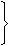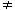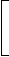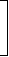# Topic: Basic Numeracy (Test 3)

Topic: Basic Numeracy
Q.1
Running at the same constant rate, 6 identical machines can produce a total of 270 bottles per minute. At this rate, how many bottles could 10 such machines produce in 4 minutes?
A. 648
B. 1800
C. 2700
D. 10800
Explaination / Solution:

Let the required number of bottles be x.

More machines, More bottles (Direct Proportion)

More minutes, More bottles (Direct Proportion)

 Machines 6 : 10:: 270 : x Time (in minutes) 1 : 46 x 1 x x = 10 x 4 x 270x = (10 x 4 x 270) (6)x = 1800.

Workspace
Report
Q.2
A boat can travel with a speed of 13 km/hr in still water. If the speed of the stream is 4 km/hr, find the time taken by the boat to go 68 km downstream.
A. 2 hours
B. 3 hours
C. 4 hours
D. 5 hours
Explaination / Solution:

Speed downstream = (13 + 4) km/hr = 17 km/hr.

 Time taken to travel 68 km downstream =68hrs = 4 hrs. 17

Workspace
Report
Q.3
A man's speed with the current is 15 km/hr and the speed of the current is 2.5 km/hr. The man's speed against the current is:
A. 8.5 km/hr
B. 9 km/hr
C. 10 km/hr
D. 12.5 km/hr
Explaination / Solution:

Man's rate in still water = (15 - 2.5) km/hr = 12.5 km/hr.

Man's rate against the current = (12.5 - 2.5) km/hr = 10 km/hr.

Workspace
Report
Q.4
Which of the following statements is not correct?
A. log10 10 = 1
B. log (2 + 3) = log (2 x 3)
C. log10 1 = 0
D. log (1 + 2 + 3) = log 1 + log 2 + log 3
Explaination / Solution:

(a) Since loga a = 1, so log10 10 = 1.

(b) log (2 + 3) = log 5 and log (2 x 3) = log 6 = log 2 + log 3log (2 + 3)log (2 x 3)

(c) Since loga 1 = 0, so log10 1 = 0.

(d) log (1 + 2 + 3) = log 6 = log (1 x 2 x 3) = log 1 + log 2 + log 3.

So, (b) is incorrect.

Workspace
Report
Q.5
If log 2 = 0.3010 and log 3 = 0.4771, the value of log5 512 is:
A. 2.870
B. 2.967
C. 3.876
D. 3.912
Explaination / Solution:

log5 512
 = log 512 log 5
 = log 29 log (10/2)
 = 9 log 2 log 10 - log 2
 = (9 x 0.3010) 1 - 0.3010
 = 2.709 0.699
 = 2709 699
= 3.876

Workspace
Report
Q.6
In order to obtain an income of Rs. 650 from 10% stock at Rs. 96, one must make an investment of:
A. Rs. 3100
B. Rs. 6240
C. Rs. 6500
D. Rs. 9600
Explaination / Solution:

To obtain Rs. 10, investment = Rs. 96.

 To obtain Rs. 650, investment = Rs.96 x 650= Rs. 6240. 10

Workspace
Report
Q.7
A man buys Rs. 20 shares paying 9% dividend. The man wants to have an interest of 12% on his money. The market value of each share is:
A. Rs. 12
B. Rs. 15
C. Rs. 18
D. Rs. 21
Explaination / Solution:

 Dividend on Rs. 20 = Rs.9 x 20= Rs. 9 . 100 5

Rs. 12 is an income on Rs. 100.Rs. 9 is an income on Rs.100 x 9= Rs. 15. 5 12 5

Workspace
Report
Q.8
A man purchased a cow for Rs. 3000 and sold it the same day for Rs. 3600, allowing the buyer a credit of 2 years. If the rate of interest be 10% per annum, then the man has a gain of:
A. 0%
B. 5%
C. 7.5%
D. 10%
Explaination / Solution:

C.P. = Rs. 3000.

 S.P. = Rs.3600 x 100= Rs. 3000. 100 + (10 x 2)

Gain = 0%.

Workspace
Report
Q.9
A trader owes a merchant Rs. 10,028 due 1 year hence. The trader wants to settle the account after 3 months. If the rate of interest 12% per annum, how much cash should he pay?
A. Rs. 9025.20
B. Rs. 9200
C. Rs. 9600
D. Rs. 9560
Explaination / Solution:

Required money= P.W. of Rs. 10028 due 9 months hence
= Rs.10028 x 100100 +12 x 912
= Rs. 9200.

Workspace
Report
Q.10
Which one of the following is not a prime number?
A. 31
B. 61
C. 71
D. 91